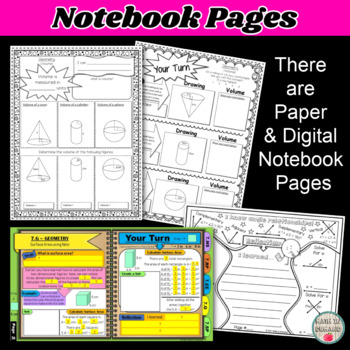# 7th Grade Math Unit 4 Geometry Curriculum7th
Subjects
Standards
Resource Type
Formats Included
• Zip
Pages
NA

### Description

This bundle includes all of my 7th Grade Math Unit 4 Resources (7.G - Geometry). There are interactive notebook pages, activities, foldables, practice worksheets, homework, warm-ups, exit slips, assessments (pre- and post-tests), vocabulary quizzes, and so much more! There are also some digital resources such as early finishers and essential questions.

All of the pre-tests and post-tests are editable. The early finishers and essential questions are given in PowerPoint. You can upload the file into Google Slides and share the link with students.

Math concepts included are:

1.) Constructing Triangles

2.) Angle Relationships

3.) Area & Perimeter of Rectangles

4.) Area & Perimeter of Triangles

5.) Area & Circumference of Circles

6.) Similar Figures

7.) Scale Drawings

8.) Volume

9.) Surface Area

Check out the PREVIEW to see more!!!

I also have other units for 7th Grade Math

Unit 1 - Number Sense

Unit 2 - Ratios & Proportional Relationships

Unit 3 - Expressions & Equations

Unit 5 - Statistics & Probability

Questions? Ask a question on the tab section or contact me by email at mathindemand@hotmail.com

Total Pages
NA
Included
Teaching Duration
N/A
Report this Resource to TpT
Reported resources will be reviewed by our team. Report this resource to let us know if this resource violates TpT’s content guidelines.

### Standards

to see state-specific standards (only available in the US).
Solve problems involving scale drawings of geometric figures, including computing actual lengths and areas from a scale drawing and reproducing a scale drawing at a different scale.
Draw (freehand, with ruler and protractor, and with technology) geometric shapes with given conditions. Focus on constructing triangles from three measures of angles or sides, noticing when the conditions determine a unique triangle, more than one triangle, or no triangle.
Describe the two-dimensional figures that result from slicing three-dimensional figures, as in plane sections of right rectangular prisms and right rectangular pyramids.
Know the formulas for the area and circumference of a circle and use them to solve problems; give an informal derivation of the relationship between the circumference and area of a circle.
Use facts about supplementary, complementary, vertical, and adjacent angles in a multi-step problem to write and solve simple equations for an unknown angle in a figure.